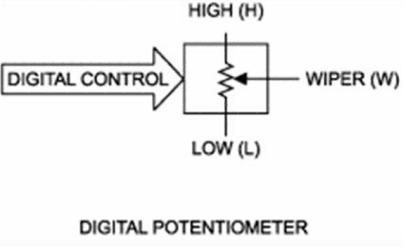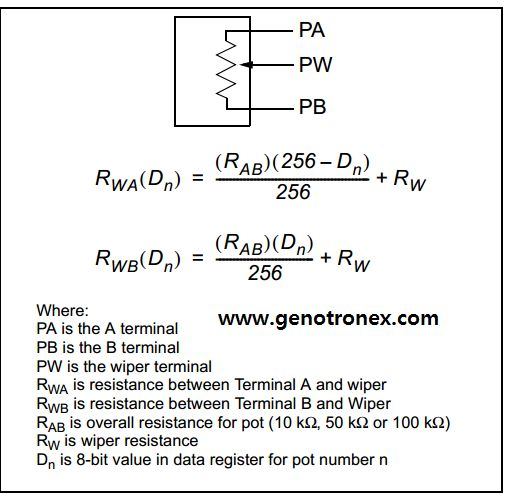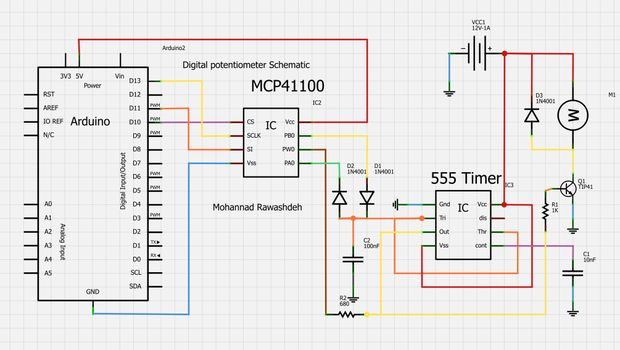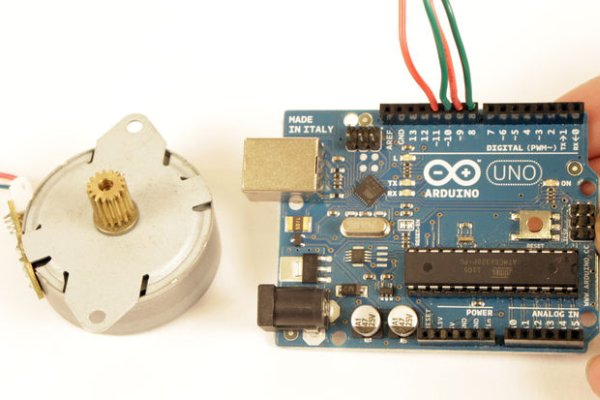# MCP41100 an Arduino Controlled PotentiometerWe know the analog potentiometer , is a three-terminal resistor with a sliding contact that forms an adjustable voltage divider .

MCP41100 an Arduino Controlled Potentiometer:

1)volume controls on audio equipment.
2) Control the amplifier Gain and offset .
3) Transducer ” displacement  transducers “.

and many Other application , But did you you want to control the resistance value by Microcontroller instead  of using analog one ?

Analog potentiometers have some problem with MCU ,  Microcontroller doesn’t have an easy way to interface with them .

The Digital Potentiometer ,  give you an ability to adjust the resistance ,allowing you to control a voltage splitter with digital signals

In this simple instructable I will show you how  to control Digital Potentiometer MCP41100 Using Arduino IDE .

Step 1: Material

we will need :

1) Arduino Board .
2)Digital Potentiometer MCP41100 .
3)Resistor 100 Ohm
4)LED
6JumperS

The pin Configuration for MCP41100 above , very easy .

Data sheet for MCP41100 here

This IC using SPI Protocol to communicate with Arduino Board .

## Step 2: Calculate the resistance .Ok , now we will look at this table , which is show who we can Calculate the Output resistance .

MCP41100 Have a sensitivity of 8bit , 256 taps for each potentiometer will taken from .

The MCP41100 Nominal resistane equal 100K ohm “Rab” and from the Datasheet , The wiper resistance is 125 ohm”Typical” ,

For example , if we write 222 to MCP41100 ,The resistance will equal :

Rwa= (100*10^3)*(256-222)/256  – 125 = 13.41K ohm .

## Step 3: Arduino Code```/*
this program taken from arduino Example .
http://www.genotronex.com
http://www.instructables.com/

This code used to control the digital potentiometer
MCP41100 connected to  arduino Board
CS >>> D10
SCLK >> D13
DI  >>> D11
PA0 TO VCC
PBO TO GND
PW0 TO led with resistor 100ohm .
*/
#include <SPI.h>
int CS= 10;
int i=0;

void setup()
{
pinMode (CS, OUTPUT);
SPI.begin();
// adjust high and low resistance of potentiometer
digitalPotWrite(0x00);
delay(1000);

// adjust  wiper in the  Mid point  .
digitalPotWrite(0x80);
delay(1000);

digitalPotWrite(0xFF);
delay(1000);
}

void loop()
{
for (i = 0; i <= 255; i++)
{
digitalPotWrite(i);
delay(10);
}
delay(500);
for (i = 255; i >= 0; i--)
{
digitalPotWrite(i);
delay(10);
}
}

int digitalPotWrite(int value)
{
digitalWrite(CS, LOW);
SPI.transfer(value);
digitalWrite(CS, HIGH);

```

## Step 4: Simple applications :Motor drive controller and LED ControllerThis Is a simple example to control the speed of DC Motor using555 timer  ,  PWM Technique

the arduino Code :

```/*
this program taken from arduino Example .
http://www.genotronex.com
This code used to control the digital potentiometer
MCP41100 connected to  arduino Board
CS >>> D10
SCLK >> D13
DI  >>> D11
PA0 TO VCC
PBO TO GND
PW0 TO led with resistor 100ohm .
*/
#include <SPI.h>
int CS= 10;

void setup()
{
pinMode (CS, OUTPUT);
SPI.begin();
// adjust high and low resistance of potentiometer
digitalPotWrite(0x00);
delay(1000);

// adjust  wiper in the  Mid point  .
digitalPotWrite(0x80);
delay(1000);

digitalPotWrite(0xFF);
delay(1000);
}

void loop()
{
for (int i = 0; i <= 255; i++)
{
digitalPotWrite(i);
delay(10);
}
delay(1000);
for (int i = 255; i >= 0; i--)
{
digitalPotWrite(i);
delay(5);
}

}

int digitalPotWrite(int value)
{
digitalWrite(CS, LOW);
SPI.transfer(value);
digitalWrite(CS, HIGH);
```

Video :

#### This Post / Project can also be found using search terms:

• mcp 41100 Arduino example
• medidas de una barra de cocina